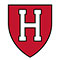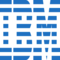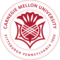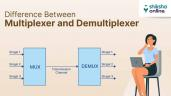An Introduction to Different Methods of Clustering In Machine Learning

# An Introduction to Different Methods of Clustering In Machine Learning

clickHere
Updated on Dec 24, 2021 10:35 IST

Clustering in Machine Learning is a technique that involves the clustering of data points. In any data set, clustering algorithms are used to classify each data point into a specific cluster. Theoretically, data points in the same cluster should have similar properties and/or characteristics, while data points in different clusters should have very different properties and/or characteristics. Clustering is an Unsupervised Machine Learning method and is a very popular statistical data analysis methodology.

Clustering has also found its way deep in data science and machine learning where it is used to cluster the data points using clustering algorithms and gain useful insights. This article covers 5 different clustering methods in machine learning, which are –“

• Hierarchical Clustering
• Partitioning Clustering
• Fuzzy Clustering
• Density-Based Spatial Clustering of Applications with Noise (DBSCAN)
• Distribution Model-Based Clustering

To learn about machine learning, read our blog – What is machine learning?

## Hierarchical Clustering

In hierarchical methods, individuals are not divided into clusters at once, but rather successive partitions are made at “different levels of aggregation or grouping”. Hierarchical Clustering is subdivided into two types –

Agglomerative clustering (bottom-up) – Clustering begins at the base of the tree, where each observation forms an individual cluster. The clusters are combined as the structure grows to converge in a single central “branch”.

Divisive clustering (top-down) – It is the opposite strategy to agglomerative clustering, which starts with all the observations contained in the same cluster, and divisions occur until each observation forms an individual cluster.

Must Read – Top 10 Machine Learning Algorithms for BeginnersEstablishing a hierarchical classification implies being able to make a series of partitions of the total set of individuals

W = {i 1, i 2,…, i N }; so that there are partitions at different levels that add (or disaggregate, if it is a divisive method) to the partitions of the lower levels.

#### Explore ML courses

The representation of the hierarchy of clusters obtained is usually carried out by means of a diagram in the form of an inverted tree called a “dendrogram”, in which the successive mergers of the branches at the different levels inform us of the successive mergers of the groups in higher-level groups (larger size, less homogeneity) successively.

You May Like to Learn About Confusion Matrix in Machine Learning

## Partitioning Clustering

Partitioning Clustering algorithms generate multiple partitions and then evaluate them according to defined criteria. They are also known as non-hierarchical since each instance is placed in exactly one of k mutually exclusive clusters.

Since only one set of clusters is the output of a typical partition cluster algorithm, the user is required to enter the desired number of clusters (usually called k). One of the most widely used partition clustering algorithms is the k-means clustering algorithm.

The user must provide the number of clusters (k) before starting and the algorithm first initializes the centers (or centroids) of the k partitions. Simply put, the k-means clustering algorithm then assigns members based on current centers and re-estimates centers based on current members.

Must Read – Statistical Methods Every Data Scientist Should Know

### How K-Means works

The algorithm works iteratively to assign each “point” (the rows of our input set form a coordinate) one of the “K” groups based on its characteristics. They are grouped based on the similarity of their features (the columns). As a result of executing the algorithm we will have:

• The “centroids” of each group will be the “coordinates” of each of the K sets that will be used to label new samples.
• Labels for the training dataset. Each label belonging to one of the K groups was formed.

The groups are defined in an organic way, that is, their position is adjusted in each iteration of the process until the algorithm converges. Once the centroids are found, we must analyze them to see what their unique characteristics are, compared to that of the other groups. These groups are the labels that the algorithm generates.

## Fuzzy Clustering

Fuzzy clustering or soft clustering methods are characterized in that each observation can potentially belong to several clusters, specifically, each observation is assigned a degree of membership to each of the clusters.

Fuzzy c-means (FCM) is one of the most widely used algorithms to generate fuzzy clustering. It closely resembles the k-means algorithm but with two differences:

• The calculation of the centroids of the clusters. The definition of centroid used by c-means is: the mean of all the observations in the data set weighted by the probability of belonging to the cluster.
• Returns for each observation the probability of belonging to each cluster.

You may also be interested in exploring:

 Popular Data Science Basics Online Courses & Certifications Popular Machine Learning Online Courses & Certifications Popular Deep Learning Online Courses & Certifications Popular Python for data science Online Courses & Certifications

## DBSCAN

Density-Based Spatial Clustering of Applications with Noise (DBSCAN) is the methodology to identify clusters following the intuitive way in which the human brain does. It identifies regions with a high density of observations separated by regions of low density.

See the following two-dimensional representation of a matrix, where the second image represents how a human eye can see the difference between monochrome and color-based clusters.

The DBSCAN algorithm needs two parameters:

Epsilon (ϵ) – It is the radius that defines the neighboring region to an observation, also called ϵ- neighborhood.

Minimum points (minPts) – Minimum number of observations within the epsilon region.

The DBSCAN algorithm is the fastest clustering method, but it is only appropriate if a very clear Search Distance can be used, and it works well with all potential clusters. This requires that all significant clusters have similar densities. DBSCAN method also allows you to use the Time Field and Search Time Range parameters to find point clusters in space and time.

Try this – Steps to Create Your Own Machine Learning Models

## Distribution Model-Based Clustering

The distribution model-based clustering method involves dividing data on the probability of how a dataset belongs to a probability distribution, where each group represents different clusters. The clustering is done basis Gaussian distribution.

Each cluster k is modeled by the normal or Gaussian distribution, defined by the below parameters –

• Μk – Mean vector
• ∑k – Covariance matrix
• An associated probability in the distribution. There is a probability of every point belonging to each cluster.

Steps involved in Clustering

Below steps are involved in data clustering –

STEP 1

Standardization – To calculate good clusters it is necessary to standardize the data. This means that you need to make your quantitative variables have a mean of 0 and a standard deviation of 1.

STEP2

Draw the matrix plot and the correlation between features – It is important to get an idea of ​​the variables that are most related to each other to know which variables can dominate the clusters. In the end, you would need to describe your quantitative variables.

STEP 3

Calculate the optimal number of clusters – We can calculate the optimal number of clusters using k-means and Gaussian mixture models for good clustering.

STEP 4

Calculate the clusters with different techniques – Different techniques like k-means, GMM or hierarchical methods can be used to interpret the clusters. You can draw conclusions basis the performance of each clustering technique.

STEP 5

Compare the clusters you have calculated – The last step is to compare the characteristics of the groups that you have created with the preferred technique selected in step 4. See if you find significant differences between groups according to the characteristics and in which variables those differences are seen. This will help you interpret the clusters that you have calculated.

## Conclusion

Clustering is an easy-to-implement methodology and is a crucial component of data mining and machine learning techniques. However, you need to take care of aspects like removing outliers in your data, cleaning the data, and ensuring that your data sets are sufficient and accurate. The applications of clustering are immense. We will discuss the application of the clustering algorithm in our next article.

Keep learning!

If you have recently completed a professional course/certification, click here to submit a review.

clickHere

Rashmi is a postgraduate in Biotechnology with a flair for research-oriented work and has an experience of over 13 years in content creation and social media handling. She has a diversified writing portfolio and aim... Read Full Bio

## Trending Data Science CoursesMachine Learning
Coursera4.6## Top Picks & New Arrivals